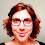## Friday, February 1, 2013

### A comment on the distribution of residuals (and data) for phylogenetic ANOVA

I get inquiries (with some regularity) about "testing for normality in phylogenetic (i.e., species) data" before phylogenetic regression or ANOVA; or about "satisfying the assumptions of parametric tests," by which is usually meant the assumption of normality.

I could probably write a whole paper about this (à la Revell 2009 or Revell 2010), but instead I'll make the simple point: we do not expect normality of the dependent (or independent, for continuous x) variables in phylogenetic data. Instead, what we do expect is multivariate normality of the residual error in y given X (or, equivalently, normality of y given X, controlling for the tree). This is actually a generally under-appreciated property of non-phylogenetic parametric statistical tests - but it is one that is entirely logical. Think: do we expect normality of human height, say, in order to fit an ANOVA model in which height depends on sex? Of course not, the response variable (height) is bimodal. ANOVA is appropriate to test for an effect of sex on height so long as the residual error in height controlling for sex is normal (and, like many such tests, may be fairly robust to mild violations of this assumption). Phylogenetic data are just a little more complicated because even after controlling for our main effects, the residual error can still be bi- or multi-modal due to phylogenetic correlations.

We can still test the parametric assumptions of our model - and I applaud those inclined to do so, as this is relatively seldom done in comparative studies. In the example below, I will first simulate data under the assumptions of the generalized phylogenetic ANCOVA; test normality of the response variable, y (it should fail) and my continuous covariate, x2 (it should fail); fit the phylogenetic ANCOVA model anyway, and then test normality of the residuals (these should fail, because the residuals are phylogenetically autocorrelated, see Revell 2009); mathematically "remove" the phylogeny, following Butler et al. (2000), and test for normality again (this time, it should pass). For normality testing, I'm using the Lilliefors (Kolmogorov-Smirnov) test, implemented in the R package nortest. A significant result means the data are not normally distributed.

> require(phytools)
> require(nlme)
> require(nortest)
> # first simulate a completely balanced tree
> tree<-compute.brlen(stree(n=128,type="balanced"))
> # now simulate a discrete character on the tree
> Q<-matrix(c(-2,1,1,1,-2,1,1,1,-2),3,3)
> rownames(Q)<-colnames(Q)<-c(0,1,2)
> mtree<-sim.history(tree,Q)
> cols<-c("white","blue","red"); names(cols)<-0:2
> plotTree(mtree,ftype="off",lwd=5)
This is the distribution of our effect on the tree.

> # now simulate data under an arbitrary ANCOVA model
> # the same principle applies to regression or ANOVA
> x1<-as.factor(mtree\$states)
> x2<-fastBM(tree,sig2=2)
> e<-fastBM(tree)
> y<-2*as.numeric(x1)+0.75*x2+e
> # is y normal? (should fail)
> lillie.test(y)

Lilliefors (Kolmogorov-Smirnov) normality test

data:  y
D = 0.1049, p-value = 0.00149

> # is x2 normal? (should fail)
> lillie.test(x2)

Lilliefors (Kolmogorov-Smirnov) normality test

data:  x2
D = 0.1113, p-value = 0.0005154

> # fit the model
> fit<-gls(y~x1+x2,data=data.frame(x1,x2,y), correlation=corBrownian(1,tree))
> fit
Generalized least squares fit by REML
Model: y ~ x1 + x2
Data: data.frame(x1, x2, y)
Log-restricted-likelihood: 40.7237

Coefficients:
(Intercept)         x11         x12          x2
1.7388578   1.8929459   3.9681291   0.8418073

Correlation Structure: corBrownian
Formula: ~1
Parameter estimate(s):
numeric(0)
Degrees of freedom: 128 total; 124 residual
Residual standard error: 0.9261019
> # are the residuals normal? (should fail)
> lillie.test(residuals(fit))

Lilliefors (Kolmogorov-Smirnov) normality test

data:  residuals(fit)
D = 0.1156, p-value = 0.0002458

> # are the residuals controlling for phylogeny normal?
> # (should pass)
> lillie.test(chol(solve(vcv(tree)))%*%residuals(fit))

Lilliefors (Kolmogorov-Smirnov) normality test

data:  chol(solve(vcv(tree))) %*% residuals(fit)
D = 0.0694, p-value = 0.1371

The basic point is that we do not expect our input data in phylogenetic ANOVA or regression to be normally distributed - just the residual error controlling both for the main effects in our model, and (importantly, because this is most often forgotten) the tree.

1.Liam,

This is super informative. However, what are we to do when the residual error controlling for the model effects and tree are NOT normal. Both my predictor and response variables are highly right-skewed; the former are also zero-inflated. PGLS seems to perform poorly, and your test (above) fails (D = 0.25158, p-value < 2.2e-16). Transforming the data before PGLS seems to perform poorly - most transformations of the zero-inflated data result in odd distributions, and residuals from PGLS with transformed data still fail your test (albeit barely, p-values around 0.004-0.01). Are there any tests incorporating a phylogenetic control that might work with these data distributions?

Thanks!

Mike

1.I don't know, but you might consider a GLMM in which you can use different link functions. This can be done incorporating the phylogeny using the package mcmcGLMM.

2.I would also love to know the answer to Mike's question please Liam.

3.This post might help:
https://www.researchgate.net/post/Why_do_the_residuals_need_to_be_normal_when_carrying_out_multi_level_modeling

Note: due to the very large amount of spam, all comments are now automatically submitted for moderation.